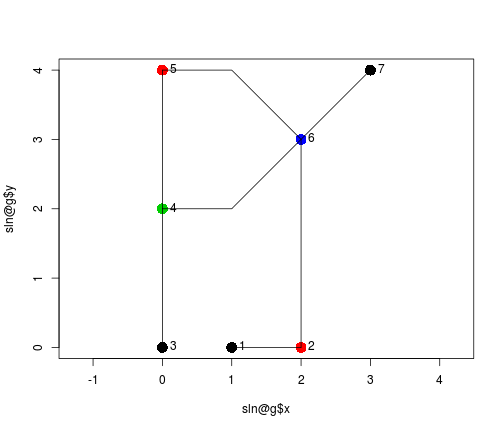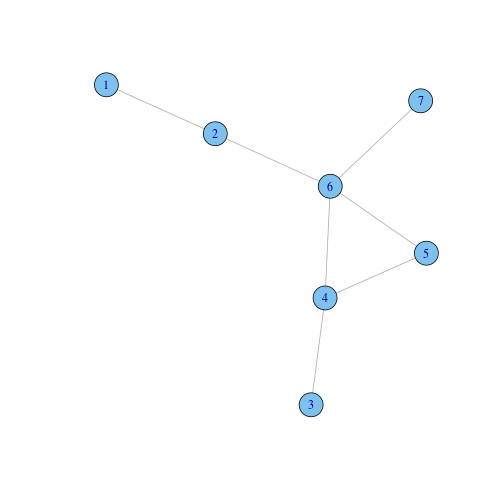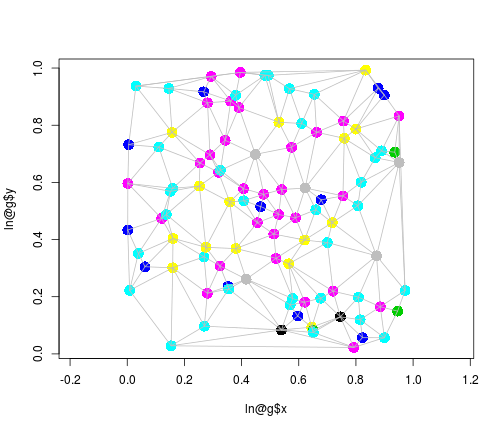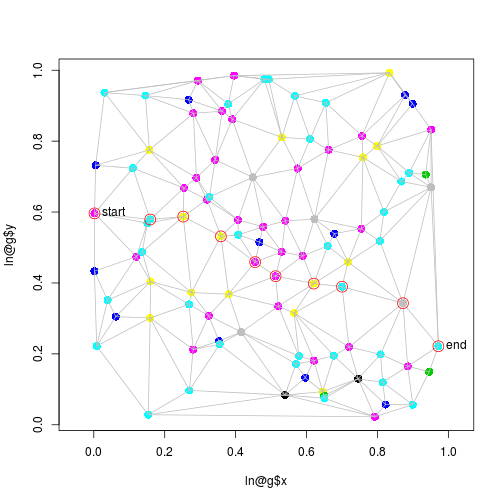# Spatial networks

A missing sp-compatible spatial data class is that for spatial networks. This document will do some simple things by combining the spatial (`SpatialLines`) classes of `sp` with the graph classes and methods from `igraph`. We will compute and plot the shortest path through a graph made of Delauney triangle edges from random points.

To start, we'll need to inport the spatial and graph packages:

``````library(sp)
library(igraph)
``````

and define the igraph class as S4, in order to reuse it in a new S4 class

``````setClass("igraph")
``````

Next, we define the `SpatialLinesNetwork` class, consisting of

• a `SpatialLines object`, containing the spatial lines representing the edges
• an `igraph` object, containing the network topology
• a neighbourhood list `nb`, containing for each vertex which edges it connets to:
``````setClass("SpatialLinesNetwork", representation(sl = "SpatialLines", g = "igraph",
nb = "list"), validity = function(object) {
stopifnot(length(object@sl) == length(E(object@g)))
stopifnot(length(object@nb) == length(V(object@g)))
})
``````

The following section creates a `SpatialLines` object, and converts it into a `SpatialLinesNetwork`. As you can see, it only accepts “simple” objects, having only one `Line` per `Lines` set. Much of what it does is figure out the common points (igraph: vertices) where line segments (igraph: edges) meet.

``````SpatialLinesNetwork = function(sl) {
stopifnot(is(sl, "SpatialLines"))
if (!is(sl, "SpatialLinesDataFrame"))
sl = new("SpatialLinesDataFrame", sl, data = data.frame(id = 1:length(sl)))
if (!all(sapply(sl@lines, length) == 1))
stop("SpatialLines is not simple: each Lines element should have only a single Line")
startEndPoints = function(x) {
firstLast = function(L) {
cc = coordinates(L)[]
rbind(cc[1, ], cc[nrow(cc), ])
}
do.call(rbind, lapply(x@lines, firstLast))
}
s = startEndPoints(sl)
zd = zerodist(SpatialPoints(s))
pts = 1:nrow(s)

# the following can't be done vector-wise, there is a progressive effect:
if (nrow(zd) > 0) {
for (i in 1:nrow(zd)) pts[zd[i, 2]] = pts[zd[i, 1]]
}
stopifnot(identical(s, s[pts, ]))

# map to 1:length(unique(pts))
pts0 = match(pts, unique(pts))
node = rep(1:length(sl), each = 2)
nb = lapply(1:length(unique(pts)), function(x) node[which(pts0 == x)])
g = graph(pts0, directed = FALSE)  # edges
nodes = s[unique(pts), ]
g\$x = nodes[, 1]  # x-coordinate vertex
g\$y = nodes[, 2]  # y-coordinate vertex
g\$n = as.vector(table(pts0))  # nr of edges
# line lengths:
sl\$length = sapply(sl@lines, function(x) LineLength(x@Lines[]))
E(g)\$weight = sl\$length
# create list with vertices, starting/stopping for each edge?  add for
# each SpatialLines, the start and stop vertex
pts2 = matrix(pts0, ncol = 2, byrow = TRUE)
sl\$start = pts2[, 1]
sl\$end = pts2[, 2]
new("SpatialLinesNetwork", sl = sl, g = g, nb = nb)
}
``````
``````l0 = cbind(c(1, 2), c(0, 0))
l1 = cbind(c(0, 0, 0), c(0, 1, 2))
l2 = cbind(c(0, 0, 0), c(2, 3, 4))
l3 = cbind(c(0, 1, 2), c(2, 2, 3))
l4 = cbind(c(0, 1, 2), c(4, 4, 3))
l5 = cbind(c(2, 2), c(0, 3))
l6 = cbind(c(2, 3), c(3, 4))
l = list(Lines(list(Line(l0)), "e"), Lines(list(Line(l1)), "a"), Lines(list(Line(l2)),
"b"), Lines(list(Line(l3)), "c"), Lines(list(Line(l4)), "d"), Lines(list(Line(l5)),
"f"), Lines(list(Line(l6)), "g"))
sl = SpatialLines(l)
sln = SpatialLinesNetwork(sl)
``````

We can plot these data in geographical space, using colour to denote the number of edges at each vertex:

``````plot(sln@g\$x, sln@g\$y, col = sln@g\$n, pch = 16, cex = 2, asp = 1)
lines(sl)
text(sln@g\$x, sln@g\$y, E(sln@g), pos = 4)
``````The plot in the graph space looks like this:

``````plot(sln@g)
``````## A larger example: shortest path through a Delauny triangulations network

We'll use Delauny triangulation from package `deldir`:

``````require(deldir)
``````
``````## Loading required package: deldir
``````
``````## deldir 0.0-22
``````

The following function converts a set of points into a `SpatialPolygons` or into a `SpatialLines` object:

``````dd <- function(x, ..., to = "polygons") {
stopifnot(is(x, "Spatial"))
cc = coordinates(x)
dd = deldir(list(x = cc[, 1], y = cc[, 2]), ...)
if (to == "polygons") {
tm = triMat(dd)
fn = function(ix) {
pts = tm[ix, ]
pts = c(pts, pts)
Polygons(list(Polygon(rbind(cc[pts, ]))), ix)
}
SpatialPolygons(lapply(1:nrow(tm), fn), proj4string = x@proj4string)
} else if (to == "lines") {
segs = dd\$delsgs
lst = vector("list", nrow(segs))
for (i in 1:nrow(segs)) lst[i] = Lines(list(Line(cc[c(segs[i, 5], segs[i,
6]), ])), i)
SpatialLines(lst, proj4string = x@proj4string)
} else stop("argument to should be polygons or lines")
}
``````

We'll generate a set of 100 points in a unit square:

``````set.seed(5432)
x = runif(100)
x = x[order(x)]
y = runif(100)
pts = SpatialPoints(cbind(x, y))
``````

Next, we'll get the `SpatialLines` object from it:

``````sl = dd(pts, to = "lines")
``````
``````##
##      PLEASE NOTE:  The components "delsgs" and "summary" of the
##      object returned by deldir() are now DATA FRAMES rather than
##      matrices (as they were prior to release 0.0-18).
##      See help("deldir").
##
##      PLEASE NOTE: The process that deldir() uses for determining
##      duplicated points has changed from that used in version
##      0.0-9 of this package (and previously). See help("deldir").
``````

and plot it:

``````plot(sl)
``````From this object, we can create a `SpatialLinesNetwork` by

``````ln = SpatialLinesNetwork(sl)
``````

when plotted, we can again use colour to denote the number of vertices connected to each edge:

``````plot(ln@g\$x, ln@g\$y, col = ln@g\$n, pch = 16, cex = 2, asp = 1)
lines(sl, col = "grey")
``````Now we compute the shortest path from the left-most point (1) to the right-most one (100):

``````sp = get.shortest.paths(ln@g, 1, 100)[]
``````

and plot it

``````plot(ln@g\$x, ln@g\$y, col = ln@g\$n, pch = 16, cex = 1.5, asp = 1)
lines(sl, col = "grey")
points(ln@g\$x[sp], ln@g\$y[sp], col = "red", cex = 2)
text(ln@g\$x[c(1, 100)], ln@g\$y[c(1, 100)], c("start", "end"), pos = 4)
``````As the edge weights are computed by `Line` lengths, this is the geographically shortest path.

I haven't figure out how to draw the shortest path by selecting the appropriate line segments; I guess I have to use

``````sp
``````
``````##     1  16  18  34  44  49  63  74  90 100
``````

which gives the node sequence, and match each pair, 1 - 16, 16 - 18 etc., to the start and end fields (which are now un-sorted!) – some challenges ahead.